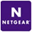# Why doesn't the amount I was charged for overage correlate with the amount of data I have in my account?

Vault On-Demand account users are charged \$5.95 per month for 5 GB of data usage, and \$0.40/GB for any usage over 5 GB.

One’s monthly usage is calculated using a "weighted average formula."  This means that you are not simply charged for the amount of data in your account on just one particular day and time - instead, we measure your usage many times throughout the month and calculate the weighted average accordingly. This is why there is sometimes a discrepancy between the usage in your account at the time your charge occurs and the overage you are charged and why the calculation most accurately reflects your activity.

For an example of how the calculation is performed, please take a look in the comments below.

0 out of 0 found this helpful
Have more questions? Submit a request

•For example, let's say you start with no data in your account at Day Zero, and we do a usage reading four times throughout your billing month (we actually take many more than four readings, but have chosen four to make this example easy to read).  Your usage report at four unique points in time is the following:

Day 7:       14 GB

Day 14:     50 GB

Day 21:     38 GB

Day 30:     7 GB

Next, we calculate the average usage between each time point. For example, the average usage between Day 7 and Day 14 = (14 + 50)/2 = 32 GB.Next, we multiply your average usage figures for each period by the fraction of the month that the respective time period represents.

For example:

Day 7 = 7/30 days, or 23% of the month = .23 * 14 GB = 3.26 GB

Day 14 = 7/30 days = .23 * 32 GB = 7.47 GB

Day 21 = 7/30 days = .23 * 44 GB = 8.87 GB

Day 30 = 9/30 days = 0.3 * 22.5 GB = 6.75 GB

Weighted average = sum of usage at four different time points = 27.75 GB

Overage charge = 27.75 GB - 5 GB included storage =  22.5 GB * \$0.40/GB = \$9.10

In conclusion, you would be charged \$9.10 in overage charges for the 30 day period described above.